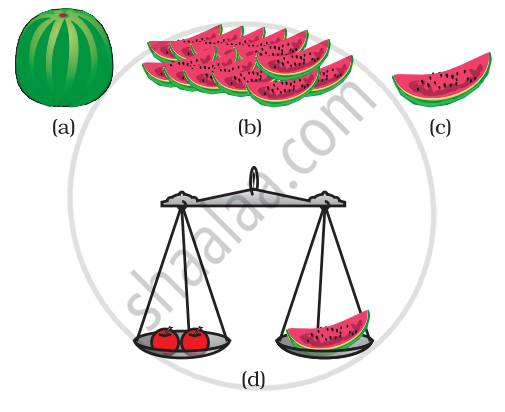# Concept of Atomic Mass:

The most remarkable concept that Dalton’s atomic theory proposed was that of the atomic mass. According to him, each element had a characteristic atomic mass. The theory could explain the law of constant proportions so well that scientists were prompted to measure the atomic mass of an atom. Since determining the mass of an individual atom was a relatively difficult task, relative atomic masses were determined using the laws of chemical combinations and the compounds formed.

Let us take the example of a compound, carbon monoxide (CO) formed by carbon and oxygen. It was observed experimentally that 3 g of carbon combines with 4 g of oxygen to form CO. In other words, carbon combines with 4/3  times its mass of oxygen. Suppose we define the atomic mass unit (earlier abbreviated as ‘amu’, but according to the latest IUPAC recommendations, it is now written as ‘u’ – unified mass) as equal to the mass of one carbon atom, then we would assign carbon an atomic mass of 1.0 u and oxygen an atomic mass of 1.33 u. However, it is more convenient to have these numbers as whole numbers or as near to whole numbers as possible. While searching for various atomic mass units, scientists initially took 1/ 16 of the mass of an atom of naturally occurring oxygen as the unit. This  was considered relevant due to two reasons:

Imagine a fruit seller selling fruits without any standard weight with him. He takes a watermelon and says, “this has a  mass equal to 12 units” (12 watermelon units or 12 fruit mass units). He makes twelve equal pieces of the watermelon and finds the mass of each fruit he is selling, relative to the mass of one piece of the watermelon. Now he sells his fruits by relative fruit mass unit (fmu), as in Fig.

(a) Watermelon, (b) 12 pieces, (c) 1/12 ofwatermelon, (d) how the fruit seller can weigh the fruits using pieces of watermelon

Similarly, the relative atomic mass of the atom of an element is defined as the average mass of the atom, as compared to 1/12th the mass of one carbon-12 atom.

Atomic masses of a few elements :

 Element Atomic mass (u) Hydrogen 1 Carbon 12 Nitrogen 14 Oxygen 16 Sodium 23 Magnesium 24 Sulphur 32 Chlorine 35.5 Calcium 40

HOW DO ATOMS EXIST?

Atoms of most elements are not able to exist independently. Atoms form molecules and ions. These molecules or ions aggregate in large numbers to form the matter that we can see, feel, or touch.

#### notes

Mass Number – denoted by ‘A’. Protons and neutrons are also called nucleons. Mass number is defined as the sum total number of protons and neutrons present in the nucleus of an atom.

In the notation for an atom, the atomic number, mass number and symbol of the element are to be written as:

Properties of mass number:

Sum of protons and neutrons provide this number of a certain element.

• It is represented by the letter A.

• Protons and Neutrons are together termed as nucleons.

• Example: Atoms of a carbon consist of 6 protons and 6 neutrons. Therefore, the mass number of Carbon is 12.

• The number of neutrons may vary in an element. However, the total number of protons is same in all atoms of an element. Therefore, the atoms of the same element with a same atomic number but a different mass number are termed as isotopes.

• Generally, atomic mass and mass numbers are two different terms and may vary slightly. In most cases, they are not the same. However, the weight of an electron is almost negligible so we can consider the atomic mass of an atom to be almost equal to its mass number.

# Concept of Atomic Mass:

The mass of an atom is concentrated in its nucleus and it is due to the protons (p) and neutrons (n) in it. (mass of electrons is negligible as compared to that of neutrons and protons)

The number (p + n) in the atomic nucleus is called the atomic mass number. Protons and neutrons are together called nucleons (mass of electrons is negligible as compared to that of neutrons and protons)

• This is commonly expressed in terms of a unified atomic mass unit (AMU).

• It can be best defined as 1/12 of the mass of a carbon-12 atom in its ground state.

If you would like to contribute notes or other learning material, please submit them using the button below.

### Shaalaa.com

Atoms and Molecules(Atomic Mass) [00:17:33]
S
0%